# Math: Elementary School: Grades 3, 4 and 5 Quiz - Division and Sharing (Questions)

This Math quiz is called 'Division and Sharing' and it has been written by teachers to help you if you are studying the subject at elementary school. Playing educational quizzes is an enjoyable way to learn if you are in the 3rd, 4th or 5th grade - aged 8 to 11.

It costs only \$12.50 per month to play this quiz and over 3,500 others that help you with your school work. You can subscribe on the page at Join Us

Division usually means sharing into equal groups. This can be related to repeated subtraction and pictures, representations, arrays or objects. These are often used to support this. Division facts can be related to the correct multiplication. For example: if a child knows that 2 x 4 = 8,
then they should recognize that 8 ÷ 4 = 2,
or 8 ÷ 2 = 4

This quiz will help children with divisions using representations and arrays.

Can you do division?

1.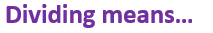[ ] Finding the total [ ] Sharing into equal groups [ ] Adding together [ ] Making numbers bigger
2.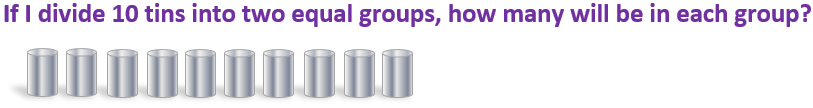[ ] 8 [ ] 11 [ ] 10 [ ] 5
3.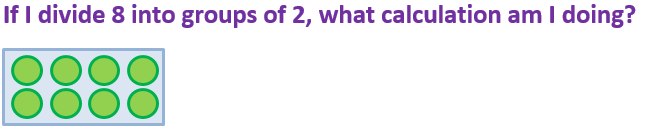[ ] 8 ÷ 2 [ ] 2 ÷ 8 [ ] 16 ÷ 8 [ ] 12 ÷ 2
4.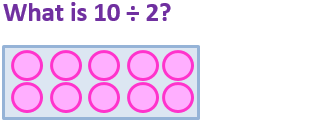[ ] 10 [ ] 5 [ ] 2 [ ] 20
5.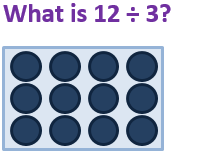[ ] 30 [ ] 12 [ ] 8 [ ] 4
6.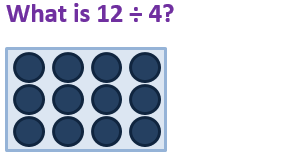[ ] 3 [ ] 4 [ ] 12 [ ] 8
7.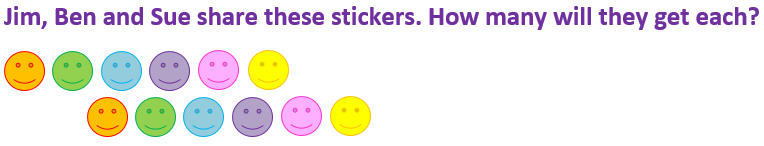[ ] 12 x 2 = 24 [ ] 12 ÷ 4 = 3 [ ] 12 x 3 = 36 [ ] 12 ÷ 3 = 4
8.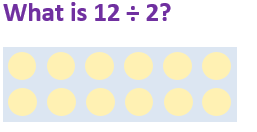[ ] 22 [ ] 16 [ ] 6 [ ] 12
9.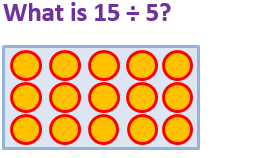[ ] 35 [ ] 5 [ ] 15 [ ] 3
10.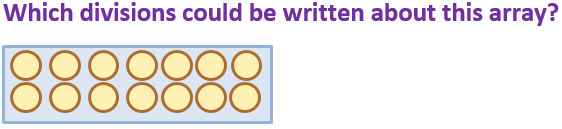[ ] 16 ÷ 8 = 2 and 16 ÷ 2 = 8 [ ] 10 ÷ 5 = 2 and 10 ÷ 2 = 5 [ ] 12 ÷ 2 = 6 and 12 ÷ 6 = 2 [ ] 14 ÷ 7 = 2 and 14 ÷ 2 = 7Math: Elementary School: Grades 3, 4 and 5 Quiz - Division and Sharing (Answers)
1.[ ] Finding the total [x] Sharing into equal groups [ ] Adding together [ ] Making numbers bigger
Dividing is the same as sharing into groups
2.[ ] 8 [ ] 11 [ ] 10 [x] 5
10 ÷ 2 = 5
3.[x] 8 ÷ 2 [ ] 2 ÷ 8 [ ] 16 ÷ 8 [ ] 12 ÷ 2
Sharing into groups of 2 is the same as dividing by 2
4.[ ] 10 [x] 5 [ ] 2 [ ] 20
10 ÷ 2 means sharing 10 into 2 equal groups, with 5 in each group
5.[ ] 30 [ ] 12 [ ] 8 [x] 4
12 shared into 3 equal groups gives 4 in each group
6.[x] 3 [ ] 4 [ ] 12 [ ] 8
12 ÷ 4 = 3 and 12 ÷ 3 = 4
7.[ ] 12 x 2 = 24 [ ] 12 ÷ 4 = 3 [ ] 12 x 3 = 36 [x] 12 ÷ 3 = 4
There are 12 stickers to be shared between 3 people, so 12 ÷ 3 = 4
8.[ ] 22 [ ] 16 [x] 6 [ ] 12
12 shared into 2 equal groups is 6
9.[ ] 35 [ ] 5 [ ] 15 [x] 3
15 shared into 5 equal groups gives 3 in each group
10.[ ] 16 ÷ 8 = 2 and 16 ÷ 2 = 8 [ ] 10 ÷ 5 = 2 and 10 ÷ 2 = 5 [ ] 12 ÷ 2 = 6 and 12 ÷ 6 = 2 [x] 14 ÷ 7 = 2 and 14 ÷ 2 = 7
There are 14 circles altogether